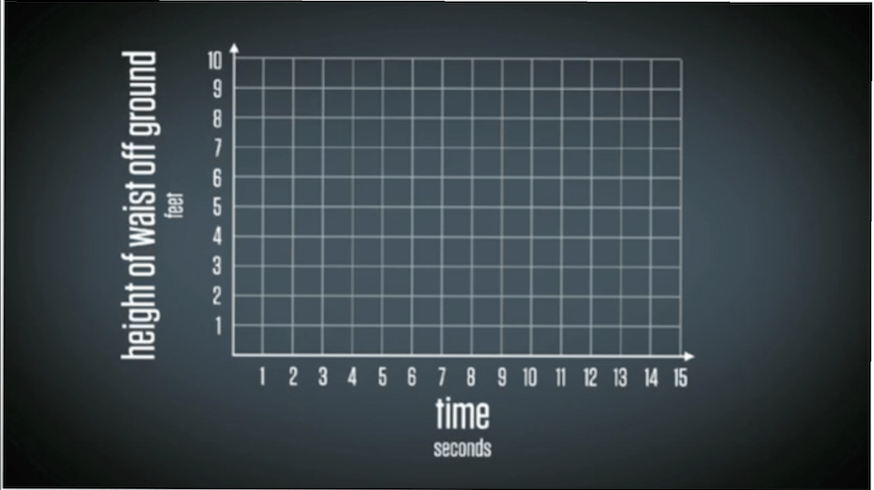# 5.5 Trigonometry & Rates of Change

Complete the following investigation.

Part 1:

1. Open the Desmos Sketch.

2. Drag the point and the tangent line along the function y = sinx.

3. For what values does the slope of the tangent line/instantaneous rate of change appear to equal zero?

4. Use the tangent line to approximate the instantaneous rate of change when x = pi/4.

5. Use the algebraic method to calculate the instantaneous rate of change at x = pi/4 to verify your estimate from #4.

Part 2:

6. Watch the video below of Dan on a swing!

7. Assuming Dan’s waist reaches a maximum height of 7 feet, and a minimum height of 2 feet. Draw a sketch of Dan’s waist height above the ground vs. time in seconds in your notes.8. Go here and watch Dan swing again and verify your graph. Create an equation to model Dan’t waist height, H(t), as a function of time, t, in seconds.

9. Find the average rate of change between the first three seconds of his swinging.

10. How fast is Dan’t waist height changing at EXACTLY 2.75 seconds?

11. Verify your answer to #10 by using the Desmos page from #1.

12. Homework questions: Page 296 #3,6,7,10## CONTACT ME